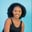Related Tags

python3

# How to use the numpy.heaviside() method for a 2D array in PythonMaria Elijah

### Overview

NumPy’s library provides a method called heaviside(), which is used to calculate the heaviside step function of the input array. This is done element by element.

Note: A list of lists can be used to create a two-dimensional (2D) array in Python.

### Syntax

numpy.heaviside(x1,x2,out=None, where=True)


### Parameters

• x1: This represents the input array.
• x2: This is array-like, and represents the value of the function when x1 is 0. x2 is commonly assumed to be 0.5. However, 0 and 1 are also used.
• out: This parameter is optional. It specifies where the result is stored.
• where: This parameter is optional. It represents the condition in which the input gets broadcasted.

Note: If the shape of x1 is not equal to the shape of x2, they must be broadcasted to a common shape.

### Return value

The numpy.heaviside() method returns the sign of each number of the input array.

### Example

The following code demonstrates how to use the numpy.heaviside() method for Python two-dimensional (2D) arrays.

# import numpy
import numpy as np
# create 2D array using np.array
x1 = np.array([[-7, -3.4 , 1.2], [2, 3 , -9.5]])
x2 = 0.5
# Compute the heaviside step function of the array
# using np.heaviside()
result = np.heaviside(x1,x2)

print(result)

### Explanation

• Line 2: We import the numpy library.
• Lines 4: We create a 2D array called x1.
• Line 5: We create a variable x2 and assign a value to it.
• Line 8: We use the np.heaviside() method to compute the heaviside step function of the input array.
• Line 10: We display the result.

RELATED TAGS

python3

CONTRIBUTORMaria Elijah
RELATED COURSES

View all Courses

Keep Exploring

Learn in-demand tech skills in half the time